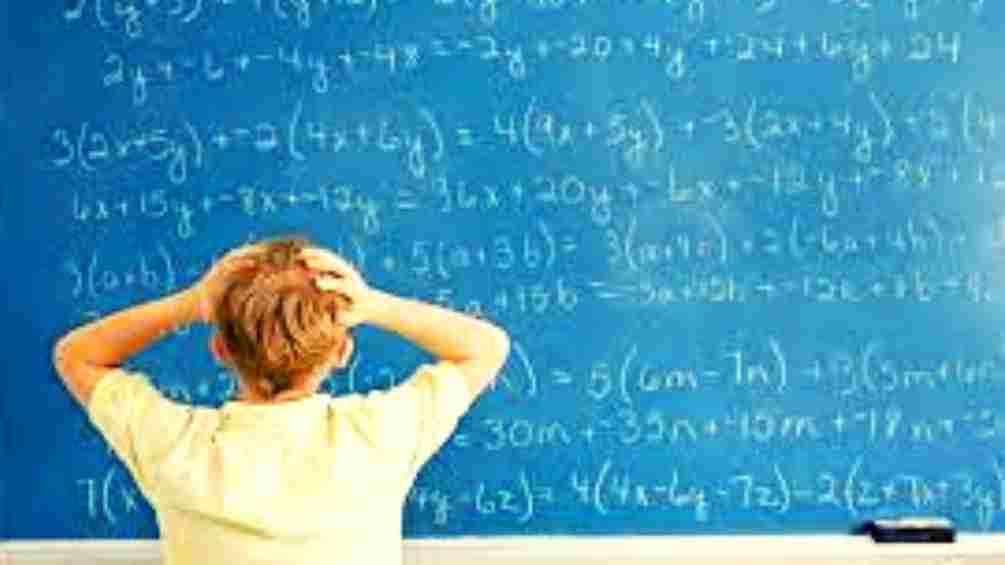# Math riddles with answers: Don't miss out these exciting math riddles. Try these fun brain teasers.

Math riddles are a fun way to recharge your mind. Try these fun math riddles.Have you ever considered math as a fun subject? Mathematics is a subject that is often not seen as a not-so-fun subject. People see the subject as something that haunts them. Why so? Well, recall your childhood days when the only subject you feared the most was mathematics. Well, it can be different for every student, but most students feared complex theorems, numbers, and formulae.

So does this mean that math is a ruthless subject that is rated by all? That's not the case. Many students like mathematics. They like the uniqueness of every question that comes. The most beautiful aspect of mathematics is that the subject has no two questions alike. Even if the concepts used in the questions are the same, there is still some uniqueness to the subject. That is what makes many students like it.

Despite the likingness, students still avoid its exams and fear the subject. This is because of the added pressure on their mind to score well in the subject. Realizing the importance of the subject in real life, society has put a lot of importance on scoring well in it. If we all remove this burden of scoring well from the students, no wonder many students will not only score well but also ace the subject.

Math riddles are nothing less than pure fun, especially when you have exciting math riddles to try out.

Try these exciting math riddles.

Math riddle 1:

How many times can you subtract five from twenty-five?

Math riddle 2:

If seven people meet each other and each shakes hands only once with each of the others, how many handshakes will there have been?

Math riddle 3:

At the time of shipping, Tom can place 10 small boxes or 8 large boxes into a carton. A total of 96 boxes were sent in one shipment. The number of small boxes was less than large boxes. What is the total number of cartons he shipped?

Math Riddle 4:

If 1=3

2=3

3=5

4=4

5=4

Then, 6=?

Math Riddle 5:

I am an odd number. Take away one letter and I become even. What number am I?

Math riddle 1:

How many times can you subtract five from twenty-five?

Answer to Riddle 1: Only once

Math riddle 2:

If seven people meet each other and each shakes hands only once with each of the others, how many handshakes will there have been?

Math riddle 3:

At the time of shipping, Tom can place 10 small boxes or 8 large boxes into a carton. A total of 96 boxes were sent in one shipment. The number of small boxes was less than large boxes. What is the total number of cartons he shipped?

Answer to Riddle 3: 11 cartons

Math Riddle 4:

If 1=3

2=3

3=5

4=4

5=4

Then, 6=?

3, as ‘six’ has three letters

Math Riddle 5:

I am an odd number. Take away one letter and I become even. What number am I?